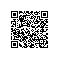# 题解 P2613 【【模板】有理数取余】

$c=\dfrac{a}{b}$   $mod$   $19260817$

---

---

$$c=\dfrac{a}{b}=a*b^{-1}$$

乘法逆元

$c=a*b^{-1}≡a*b^{p-2}$   $mod$   $p$

---

$0≤a,b≤10^{10001}$

---

---

#include<iostream>
#include<cstdio>
#include<cstring>
#include<algorithm>
using namespace std;
const int p=19260817;
int a;
int b;
char a1;
char b1;
int l1,l2;
int len1,len2;
long long x,y;

long long pow2(long long a,long long b)
{
long long res=1;
for(;b;b>>=1,a=a*a%p) if(b&1) res=res*a%p;
return res%p;
}

void calculet_1()
{
long long num=0;
for(int i=len1;i<=len1+8;i++)
num*=10,num+=a[i];

num%=p;
for(int i=len1+8;i>=len1;i--)
{
int now=num%10;num/=10;
a[i]=now;
}

for(int i=0;i<=8;i++) if(a[len1+i]!=0){len1+=i;break;}
}

void calculet_2()
{
long long num=0;
for(int i=len2;i<=len2+8;i++)
num*=10,num+=b[i];
num%=p;
for(int i=len2+8;i>=len2;i--)
{
int now=num%10;num/=10;
b[i]=now;
}

for(int i=0;i<=8;i++) if(b[len2+i]!=0){len2+=i;break;}
}

signed main()
{
//    freopen("testdata.in","r",stdin);
//    freopen("1.out","w",stdout);

scanf("%s",a1);
scanf("%s",b1);
//    printf("%s\n",b1);
l1=strlen(a1);
l2=strlen(b1);//输入以及处理数据。

for(int i=0;i<l1;i++)
a[i]=a1[i]-'0';
for(int i=0;i<l2;i++)
b[i]=b1[i]-'0';//将char 变int（个人不习惯用char做运算）

while(l1-len1>=10) calculet_1();
while(l2-len2>=10) calculet_2();//计算，我是从高位到低位依次减的，可以省时间。

for(int i=len1;i<l1;i++) x*=10,x+=a[i];
for(int i=len2;i<l2;i++) y*=10,y+=b[i];
x%=p;y%=p;//计算取模之后的值。

//    printf("%lld\n",y);
if(x==0){puts("0");return 0;}
if(y==0){puts("Angry!");return 0;}//特判

long long ans=pow2(y,p-2);
//    printf("%lld\n",ans);
ans=(ans*x)%p;

printf("%lld",ans);//计算答案和输出
return 0;//程序拜拜
}使用钉钉扫一扫加入圈子
+ 订阅